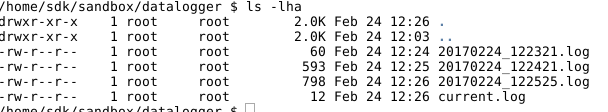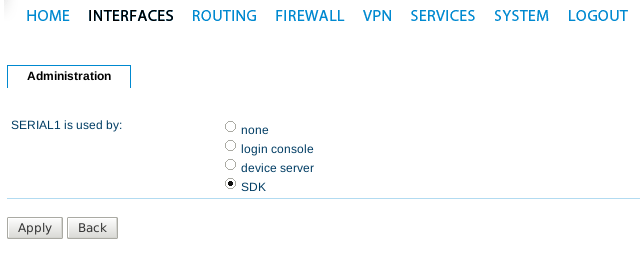# Serial Datalogger

This Script can be used to a read from the serial interface and store the data in a file. It will create new files periodicly with a timestamp in the file name to have smaller files to handle. You can combine this with the uploader.are script from the Connection Statistics Page to upload the files to a ftp server.

The Storage folder can befound via scp at /home/sdk/sandbox/## Configuration

Please set the serial-interface into “SDK”Starting in Line 115 you will find a few lines with configurationsparameter for the directory to store the files in, the new file intervall and the serial port.

```l = new logger("/datalogger/");
l.logfileIntervall=60; //create a new file every minute

// Setting for 115200 8N1 NHW Flow Control
DEV     = "SERIAL1";
BAUD    =  115200;
DATABIT = 8;
STOPBIT = 1;
PARITY  = 0;
FLOWCTL = 0;
READ_TIMEOUT = 5; /* seconds */```

## Usage

Combine the script with the eventbased trigger “sdk-startup” into a job

## The Script

```/* DESC: A Script that can be used for a longtime serial port data logging of a NetModule Wireless Router.
* The upper part of the script is the logger template.
* The lower part is the real programm, where you describe the settings and workflow.
* Copyright (C) 2014 NetModule AG, Switzerland
*/

template logger

{

workfile="current.log";
currentFilename="";
currentLogStart=0; // the current logfile was startet at this time
logfileIntervall=3600; //new logfile every # seconds
//due to the fact that we cannot see the amount of free space in the  sdk
// we need to improvise and add up the current filesizes
currentLogSize=0; //in Bytes
maxLogSize=15000000;  //in Bytes
freespace=1000000; //in Bytes
# give the constructor the absolute path to store the Loggs into
void logger(string path) {

dh=opendir(path);
if(dh == -1) {
if ( mkdir(path,0666) == -1 ) {
nb_syslog("Could not create dir: %s. Exiting",path);
exit(1);
} else {
nb_syslog("Created Logging directory: %s",path);
}
} else {
closedir(dh);
}
this.path = path;
this.fields = mkarray();
this.currentFilename=strftime("%Y%m%d_%H%M%S_start.log",localtime(time()));

}

int jiffy()
{
sys = sysinfo();
u = struct_get(sys, "uptime");

if (is_void(u) || u < 1) {
return 0;
} else {
return u;
}
}

int newLogfile() {

// check if file if we have a work file an rename it to currentFile
fd=fopen(sprintf("%s%s", this.path,this.workfile),"r" );
if(fd) {
// we have a current workfile lets rename it
fclose(fd);
if(!rename(sprintf("%s%s",this.path,this.workfile), sprintf("%s%s",this.path,this.currentFilename))){
nb_syslog("Could not rename %s to %s, %s will be deleted",this.workfile, this.currentFilename,this.workfile);
remove(sprintf("%s%s",this.path,this.workfile));
return 1;
}

}
//set new current Filename as we stored the old one away
this.currentFilename=strftime("%Y%m%d_%H%M%S.log",localtime(time()));
this.currentLogStart=this.jiffy();
// create nee workfile
fd=fopen(sprintf("%s%s", this.path,this.workfile),"a" );
if (fd < 0 ) {
nb_syslog("could not open file %s%s", this.path, this.workfile);
}
fclose(fd);

return 0;
}

fd=fopen(sprintf("%s%s", this.path,this.workfile),"a" );
if (fd < 0 ) {
nb_syslog("could not open file %s%s", this.path, this.workfile);
}

if(!fwrite(fd,sprintf("%s",line))) {
nb_syslog("writing of line to file: %s%s:", this.path,this.workfile);
fclose(fd);
return -1;
} else {
fclose(fd);
return 0;
}

}

int logNow(string serialdata)  {

nb_syslog("writing of line to file failed: %s%s:", this.path,this.workfile);
return -1;
} else {
return 0;
}

}

} // of template logger

//###########################################################################################
//###########################################################################################
//#################     The Real Rutime        ##############################################
//###########################################################################################
//###########################################################################################

l = new logger("/datalogger/");
l.logfileIntervall=60; //create a new file every minute

// Setting for 115200 8N1 NHW Flow Control
DEV     = "SERIAL1";
BAUD    =  115200;
DATABIT = 8;
STOPBIT = 1;
PARITY  = 0;
FLOWCTL = 0;
READ_TIMEOUT = 5; /* seconds */

l.newLogfile();
/* check serial port config */
st = nb_config_get("serial.0.status");
if (st != "2") {
nb_syslog("Serial port is not enabled for us. Please set Interface->Serial to SDK");
exit(1);
}

/* set attributes */
ret = nb_serial_setattr(DEV, BAUD, DATABIT, STOPBIT, PARITY, FLOWCTL);

if (ret != 0) {
nb_syslog("Could not set serial attributes: %i \n",ret);
exit(1);
}

/* open serial port */
fd = nb_serial_open(DEV);
if (fd < 0) {
nb_syslog("Unable to open %s\n", DEV);
exit(1);
}

// thats the main loop
while (true) {
// wait for serial input
if (rc == -1) {
nb_syslog("ERROR: select failed\n");
} else if (rc == 0) {
} else {
if (strlen(msg)  > 0 ) {
l.logNow(msg);
}
}
// lets see if we need a new logfile
if (l.currentLogStart+l.logfileIntervall<l.jiffy()) {
l.newLogfile();
}
}
close(fd);

exit(0);```

### Page Tools